# Affine space

The 2-dimensional plane, well-known from elementary Euclidean geometry, is an example of an affine space. Remember that in elementary geometry none of the points in the plane is special—there is no origin. A real n-dimensional affine space is distinguished from the vector space$\mathbb{R}^n$ by having no special point, no fixed origin.

From elementary geometry we know that any two points in a plane (a collection of infinitely many points) can be connected by a line segment. If the points P and Q in a plane are ordered with P before Q, the line segment connecting the two becomes an arrow pointing from P to Q. This arrow can be mapped onto a vector, the difference vector, denoted by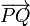$\overrightarrow{PQ}$. If all arrows in a plane can be mapped onto vectors of a 2-dimensional vector space V2, called the difference space, the plane is an affine space of dimension 2, denoted by A2. Arrows that are mapped onto the same vector in the difference space are said to be parallel, they differ from each other by translation.

In elementary analytic geometry, the map of arrows onto vectors is almost always defined by the choice of an origin O, which is a point somewhere in the plane. Clearly, an arbitrary point P is the head of an arrow with tail in the origin and corresponding with the unique difference vector$\overrightarrow{OP}$. All arrows with tail in O are mapped one-to-one onto a 2-dimensional difference space V2, with the vector addition in V2 in one-to-one correspondence with the parallelogram rule for the addition of arrows in the plane.

Usually one equips the difference space with an inner product, turning it into an inner product space. Its elements have well-defined length, namely, the square root of the inner product of the vector with itself. The distance between any two points P and Q may now be defined as the length of$\overrightarrow{PQ}$ in V2. A two-dimensional affine space, with this distance defined between the points, is the Euclidean plane known from high-school geometry.

Upon formalizing and generalizing the definition of an affine space, we replace the dimension 2 by an arbitrary finite dimension n and replace arrows by ordered pairs of points ("head" and "tail") in a given point space A. Briefly, A is an affine space of dimension n if there exists a map of the Cartesian product, A × A onto a vector space of dimension n. This map must satisfy certain axioms that are treated in the next section. If the dimension needs to be exhibited, we may write An for the affine space of dimension n.

## Formal definition

We will restrict the definition to vector spaces over the field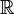$\mathbb{R}$ of real numbers.

Let V be an n-dimensional vector space and A a set of elements that we will call points. Assume that a relation between points and vectors is defined in the following way:

1. To every ordered pair P, Q of A there is assigned a vector of V, called the difference vector, denoted by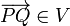$\overrightarrow{PQ} \in V$.
2. To every point P of A and every vector$\vec{v}$ of V there exists exactly one point Q in A, such that$\overrightarrow{PQ} = \vec{v}$.
3. If P, Q, and R are three arbitrary points in A, then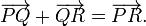$\overrightarrow{PQ} + \overrightarrow{QR} = \overrightarrow{PR}.$

If these three postulates hold, the set A is an n-dimensional affine space with difference space V.

Two immediate and important consequences are:\begin{align} \overrightarrow{PP} + \overrightarrow{PR} &= \overrightarrow{PR} &\Longrightarrow\quad \overrightarrow{PP} = \vec{0}.\qquad \\ \overrightarrow{PQ} + \overrightarrow{QP} &= \vec{0}\qquad &\Longrightarrow\quad \overrightarrow{PQ} = -\overrightarrow{QP}. \\ \end{align}

Lemma 1: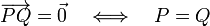$\overrightarrow{PQ} = \vec{0} \quad \Longleftrightarrow\quad P = Q$.

Proof:   If the points coincide,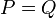$\,P = Q$, we just saw that the difference vector is the zero vector. Conversely, assume that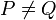$\,P \ne Q$   and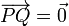$\overrightarrow{PQ} = \vec{0}$. Then for an arbitrary point$\,R$,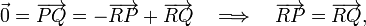$\vec{0} = \overrightarrow{PQ} = -\overrightarrow{RP} + \overrightarrow{RQ} \quad\Longrightarrow\quad \overrightarrow{RP} = \overrightarrow{RQ},$

which implies that the same vector in V connects$\,R$ in A with two different points, which by postulate 2 is forbidden.

## Parallelogram law

Consider four points in A:   P1, P2, Q1, and Q2. Assume that the following difference vectors are equal,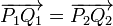$\overrightarrow{P_1Q_1} = \overrightarrow{P_2Q_2}$

then we may exchange Q1 and P2,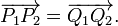$\overrightarrow{P_1P_2} = \overrightarrow{Q_1Q_2}.$

See the figure for a concrete example in which the four points form a parallelogram.

Proof:   Subtract the following equations:\begin{align} \overrightarrow{P_1P_2} &= \overrightarrow{P_1Q_2} - \overrightarrow{P_2Q_2}\\ \overrightarrow{Q_1Q_2} &= \overrightarrow{P_1Q_2} - \overrightarrow{P_1Q_1}.\\ \end{align}

This gives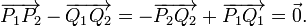$\overrightarrow{P_1P_2} - \overrightarrow{Q_1Q_2} = - \overrightarrow{P_2Q_2} + \overrightarrow{P_1Q_1} = \vec{0}.$

## Position vector

Choose a fixed point O in the affine space A, an origin. Every point P is uniquely determined by the vector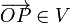$\overrightarrow{OP} \in V$. Indeed, suppose that there is another point Q such that$\overrightarrow{OP} = \overrightarrow{OQ}$, it then follows from lemma 1 that P = Q.

The vector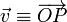$\vec{v}\equiv\overrightarrow{OP}$ is the position vector of P with respect to O. After choosing O every point P in A is uniquely identified by its corresponding position vector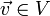$\vec{v} \in V$.

Choice of another origin O gives a translation of$\vec{v}$ by$-\vec{t} \equiv -\overrightarrow{OO\;'}$, for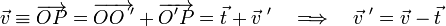$\vec{v} \equiv \overrightarrow{OP} = \overrightarrow{OO\;'}+ \overrightarrow{O'P} = \vec{t} + \vec{v}\;' \quad\Longrightarrow\quad \vec{v}\;' = \vec{v} - \vec{t}$

with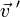$\vec{v}\;'$ the position vector of P with respect to O .

## Affine coordinate systems

An affine coordinate system$\mathbb{B} \equiv (O; \,\vec{e}_1, \vec{e}_2,\ldots, \vec{e}_n)$

consists of an origin O in A and a basis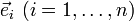$\vec{e}_i\,\, (i=1,\ldots,n)$   of the difference space V. Then every point P in A determines a system of n real numbers xi (i = 1, ..., n) by$\overrightarrow{OP} = \sum_{i=1}^n \, x_i\, \vec{e}_i \in V.$

The numbers xi (i = 1, ..., n) are the affine coordinates of P with respect to the given coordinate system. Note that O has the coordinates xi = 0.

Consider now two different affine coordinate systems,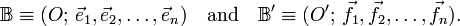$\mathbb{B} \equiv (O; \,\vec{e}_1, \vec{e}_2,\ldots, \vec{e}_n)\quad \hbox{and}\quad\mathbb{B}' \equiv (O'; \,\vec{f}_1, \vec{f}_2,\ldots, \vec{f}_n).$

Write$\vec{f}_i = \sum_{j=1}^n \vec{e}_j \, A_{ji} \quad\hbox{and}\quad \overrightarrow{OO'} = \sum_{i=1}^n t_i\, \vec{e}_i.$

The matrix (Ai j) transforms the one basis of V into the other, hence it is a square regular (invertible) matrix. The real numbers ti are the affine coordinates of O relative to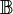$\mathbb{B}$.

Express a fixed point P with respect to$\mathbb{B}$ and$\mathbb{B}'$,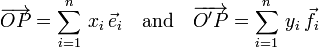$\overrightarrow{OP} = \sum_{i=1}^n \, x_i\, \vec{e}_i\quad\hbox{and}\quad \overrightarrow{O'P} = \sum_{i=1}^n \, y_i\, \vec{f}_i$

Insert into the second equation$\overrightarrow{O'P} = \overrightarrow{OP} -\overrightarrow{OO'}$

and express$\vec{f}_i$ in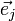$\vec{e}_j$ , then$\sum_{i=1}^n y_i\, \vec{f}_i = \sum_{j=1}^n (x_j - t_j) \vec{e}_j= \sum_{j=1}^n \vec{e}_j \sum_{j=1}^n \, A_{ji}\, y_i,$

so that the transformation from the one affine coordinate system to the other is,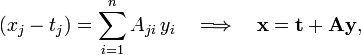$(x_j-t_j) = \sum_{i=1}^n A_{ji}\, y_i \quad \Longrightarrow\quad \mathbf{x} = \mathbf{t} + \mathbf{A} \mathbf{y},$

where we introduced bold lowercase letters for real column-vectors (stacks of n real numbers) and the boldface capital indicates an n × n matrix. Inversion of the non-singular (regular) matrix (Ai j) gives the inverse transformation,$y_j = \sum_{i=1}^n (A^{-1})_{ji}\, (x_i-t_i) \quad \Longrightarrow\quad \mathbf{y} = \mathbf{A}^{-1} \,(\mathbf{x} - \mathbf{t}).$

## Affine maps

Let PP′ be a mapping of the affine space A into itself; if the map satisfies the following two conditions, it is an affine map.

(i) Conserve "parallelism". (In the Euclidean plane this condition implies that sets of mutually parallel arrows are mapped onto sets of mutually parallel arrows. Note, however, that in general the mapped arrows are not parallel to the original arrows):$\overrightarrow{P_1 Q_1} = \overrightarrow{P_2 Q_2}\quad \Longrightarrow\quad \overrightarrow{P'_1 Q'_1} = \overrightarrow{P'_2 Q'_2}$

(ii) The map is linear in the difference space. That is, the map φ: VV defined by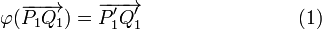$\varphi(\overrightarrow{P_1 Q_1}) = \overrightarrow{P'_1 Q'_1} \qquad\qquad\qquad\qquad(1)$

is linear. A translation is an affine map with φ the identity operation,$\varphi(\overrightarrow{P_1 Q_1})\equiv \overrightarrow{P'_1 Q'_1} = \overrightarrow{P_1 Q_1}.$

Here the origin and the image are parallel.

Given two points O and O′ and a linear map φ: VV. There exists exactly one affine map that sends O into O′ and induces φ on V. This is the map$\overrightarrow{OP\,'} = \overrightarrow{OO\,'} + \varphi(\overrightarrow{OP}) ,$

because (see Fig. 1),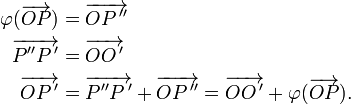\begin{align} \varphi(\overrightarrow{OP}) &= \overrightarrow{OP\,''}\\ \overrightarrow{P''P\,'} &= \overrightarrow{OO\,'}\\ \overrightarrow{OP\,'} &= \overrightarrow{P''P\,'} + \overrightarrow{OP\,''} = \overrightarrow{OO\,'} + \varphi(\overrightarrow{OP}) . \end{align}

Note: since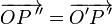$\overrightarrow{OP\,''} = \overrightarrow{O'P\,'}$ by the parallelogram law (see above), the map φ may also be defined as$\varphi(\overrightarrow{OP}) = \overrightarrow{O'P\,'},$

as was done in Eq. (1).

Choose a basis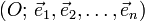$(O; \,\vec{e}_1, \vec{e}_2,\ldots, \vec{e}_n)$

then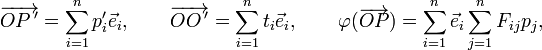$\overrightarrow{OP\,'} = \sum_{i=1}^n p'_i \vec{e}_i, \qquad \overrightarrow{OO\,'} = \sum_{i=1}^n t_i \vec{e}_i, \qquad \varphi(\overrightarrow{OP}) = \sum_{i=1}^n \vec{e}_i\sum_{j=1}^n F_{ij} p_j,$

from which follows the matrix-vector expression for an affine map,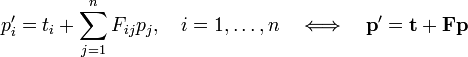$p'_i = t_i +\sum_{j=1}^n F_{ij} p_j, \quad i=1,\ldots,n \quad\Longleftrightarrow\quad \mathbf{p}' = \mathbf{t} + \mathbf{F} \mathbf{p}$

Often one writes the last expression for an affine map with the aid of a square (n+1) × (n+1) matrix that contains F on the diagonal and that is augmented with the translation vector t and the number 1,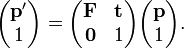$\begin{pmatrix} \mathbf{p}' \\ 1 \end{pmatrix} = \begin{pmatrix} \mathbf{F} & \mathbf{t} \\ \mathbf{0} & 1 \\ \end{pmatrix} \begin{pmatrix} \mathbf{p} \\ 1 \end{pmatrix} .$

## Euclidean space

Let A be an affine space with difference space V on which a positive-definite inner product is defined. Then A is called a Euclidean space. The distance between two point P and Q is defined by the length ,$\rho(P,Q) = |\overrightarrow{PQ}| \equiv \left(\overrightarrow{PQ},\; \overrightarrow{PQ}\right)^{1/2}$

where the expression between round brackets indicates the inner product of the vector with itself. It follows from the properties of the real inner product that the distance has the usual properties,

1. ρ(P,Q) ≥ 0 and ρ(P,Q) = 0 if and only if P = Q
2. ρ(P,Q) = ρ(Q,P)
3. ρ(P,Q) ≤ ρ(P,R) + ρ(R,Q)

A rigid motion of a Euclidean space is an affine map which preserves distances. The linear map φ on V is then a rotation. Conversely, given a rotation φ and two points P and P′ then there exists exactly one rigid motion which sends P into P′ and induces φ on V.

## Notes and references

1. V. I . Arnold, Mathematical Methods of Classical Mechanics, translated from the Russian by K. Vogtmann and A. Weinstein. Springer, New York (1978).
2. Sometimes it is stated: "the arrow is a vector", but in the present context it is necessary to carefully distinguish arrows from vectors.
3. Recall that φ is linear when$\varphi(a\vec{v} +b \vec{w}) = a \varphi(\vec{v}) + b \varphi(\vec{w}), \qquad \vec{v}, \vec{w} \in V, \quad a,b\in \mathbb{R}.$
4. The computer language PostScript calls the augmented matrix (for n = 2) the "current transformation matrix" (CTM).
• A. Lichnerowicz, Elements of Tensor Calculus, Translated from the French by J. W. Leech and D. J. Newman, Methuen (London) 1962.
• W. H. Greub, Linear Algebra, 2nd edition, Springer (Berlin) 1963.